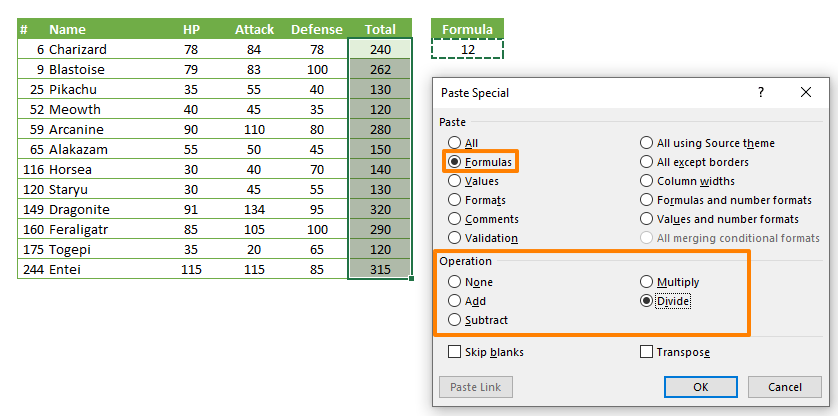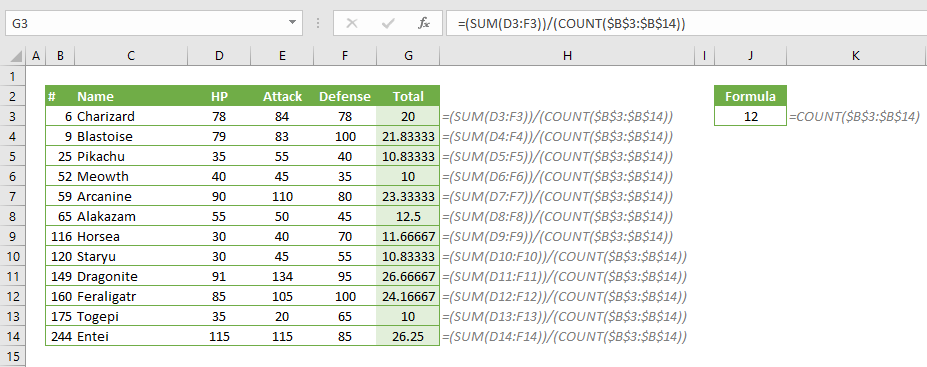In addition to using Paste Special to evaluate numbers with operators, you can also use this feature to modify formulas. Formulas can be bound to other formulas or named ranges with the use of mathematical operators. In this guide, we’re going to show how to use Paste Special to modify formulas in Excel.

## Paste Special Basics

Paste Special dialog can be accessed from the right-click context menu, or by pressing the Ctrl + Alt + V key combination (instead of just Ctrl + V) when pasting data.The dialog contains all available paste methods. The ones we are going to be needing for the purpose of this article are under the Operation section.Paste Special supports 4 main operations:

• Subtract
• Multiply
• Divide

You can select only one operation at a time when pasting. Copied cell’s value or formula will be translated into the target cells based on the selected operation. Let’s see this functionality on an example.

## How to use Paste Special to modify formulas by another formula set

Let’s say we have a set of formulas that calculate the sum of values in their row (e.g. =SUM(D3:F3)), and we want to divide these formulas by another formula in a different cell, e.g. =COUNT(\$B\$3:\$B\$14).1. Copy the cells that contains the formulas you want to add
2. Select the range of formulas you want to update
3. Press Ctrl + Alt + V to open the Paste Special dialog
4. Select Formulas and the Operation you want to apply (We selected Divide)
5. Click OKYou will see that all formulas in the target range are updated with the selected operation and the copied formula. For example, =(SUM(D3:F3))/(COUNT(\$B\$3:\$B\$14)).## How to use Paste Special to modify formulas by a variable

This scenario is useful for when you want to apply a variable modifier into the formulas. If the modifier is a static value (doesn’t contain any formulas), Paste Special copies only the value of the cell - not its reference. Thus, the modifier remains static.

To avoid this, use the following workaround:

1. Create a helper cell that references the modifier cell. For example, if your modifier resides in J3, the helper formula should be =\$J\$3. Do not forget to use absolute reference. Otherwise, reference will shift after copying.
2. Copy the cell that contains the formulas you want to add
3. Select the range of formulas you want to update
4. Press Ctrl + Alt + V to open the Paste Special dialog
5. Select Formulas and the Operation you want to apply (We selected Multiply)
6. Click OKLet’s see the how Excel modifies the formulas: